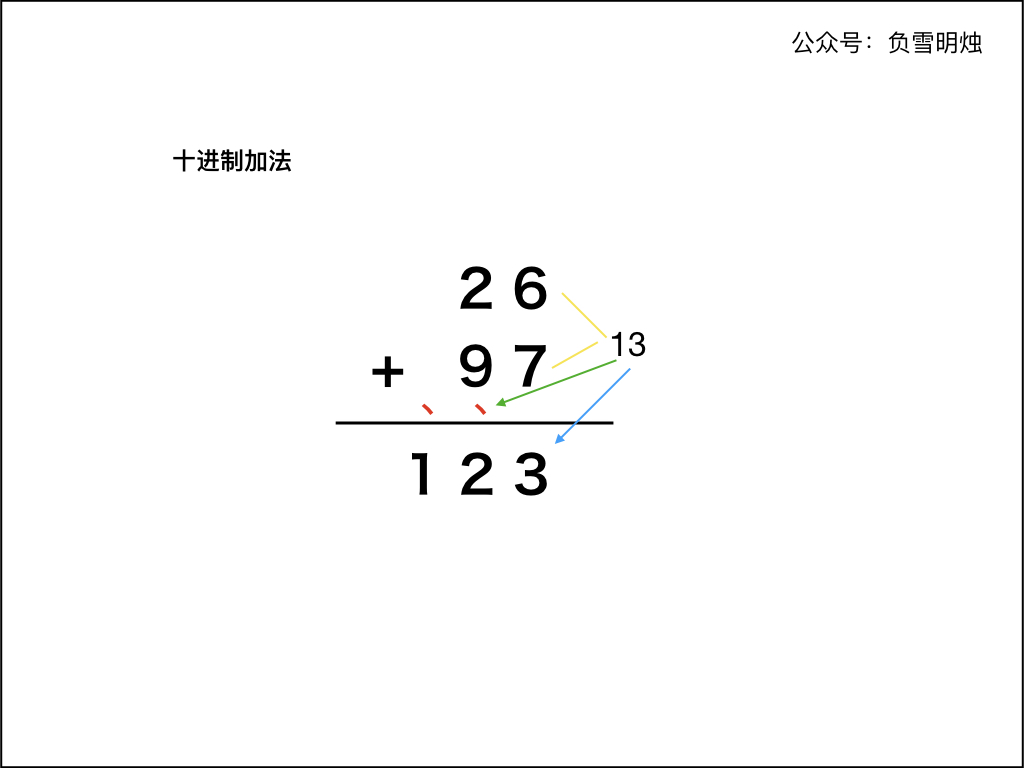# 66. Plus One 加一

• 作者： 负雪明烛
• id： fuxuemingzhu
• 个人博客： http://fuxuemingzhu.cn/
• 公众号：负雪明烛
• 本文关键词：Leetcode, 力扣，加法，两数之和，求加法，数组，Python, C++, Java

@TOC

[LeetCode]

Total Accepted: 99274 Total Submissions: 294302 Difficulty: Easy

# # 题目描述

Given a non-empty array of digits representing a non-negative integer, plus one to the integer.

The digits are stored such that the most significant digit is at the head of the list, and each element in the array contain a single digit.

You may assume the integer does not contain any leading zero, except the number 0 itself.

Example 1:

Input: [1,2,3]
Output: [1,2,4]
Explanation: The array represents the integer 123.


Example 2:

Input: [4,3,2,1]
Output: [4,3,2,2]
Explanation: The array represents the integer 4321.


# # 解题方法

## # 数九

public class Solution {
public int[] plusOne(int[] digits) {
for(int i=digits.length-1; i>=0; i--){
if(digits[i]==9){
digits[i]=0;
continue;
}else{
digits[i]+=1;
break;
}
}

if(digits==0){
int[] answer=new int[digits.length + 1];
}else{
return digits;
}
}
}


AC:0ms

## # 采用进位

### # 十进制加法1. 两个「加数」的右端对齐；
2. 从最右侧开始，依次计算对应的两位数字的和。如果和大于等于 10，则把和的个位数字计入结果，并向前面进位。
3. 依次向左计算对应位置两位数字的和，如果有进位需要加上进位。如果和大于等于 10，仍然把和的个位数字计入结果，并向前面进位。
4. 当两个「加数」的每个位置都计算完成，如果最后仍有进位，需要把进位数字保留到计算结果中。

### # 在实现中需要注意的有：

1. 不可以把 digits 先转化成 int 型数字再求和，因为可能溢出
2. 另一个「加数」是 adder ，初始化为 ，以后都是
3. 两个「加数」的长度可能不同；
4. 在最后，如果进位 carry 不为 0，那么最后需要计算进位

### # 方法：循环

#### # 代码中的巧妙之处：

1. while (p1 >= 0 || adder > 0 || carry > 0)含义：
1. digitsadder 只要有一个没遍历完，那么就继续遍历；
2. 如果字符串 digitsadder 都遍历完了，但是最后留下的进位 carry != 0，那么需要把进位也保留到结果中。
2. 取当前位置的数字的时候，如果 digits 已经遍历完了（即 ），则认为 digits 的对应位置是

Java 语言的代码如下：

class Solution {
public int[] plusOne(int[] digits) {
int N = digits.length;
List<Integer> resultList = new ArrayList<>();
int p1 = N - 1;
int carry = 0;
int adder = 1;
while (p1 >= 0 || adder > 0 || carry > 0) {
int num = p1 >= 0 ? digits[p1] : 0;
int sum = num + adder + carry;
carry = sum >= 10 ? 1 : 0;
sum = sum >= 10 ? sum - 10 : sum;
p1 --;
}
int[] res = new int[resultList.size()];
for (int i = 0; i < resultList.size(); ++i) {
res[i] = resultList.get(resultList.size() - i - 1);
}
return res;
}
}


C++ 代码如下：

class Solution {
public:
vector<int> plusOne(vector<int>& digits) {
const int N = digits.size();
vector<int> res;
int p1 = N - 1;
int carry = 0;
int adder = 1;
while (p1 >= 0 || adder > 0 || carry > 0) {
int num = p1 >= 0 ? digits[p1] : 0;
int sum = num + adder + carry;
carry = sum >= 10 ? 1 : 0;
sum = sum >= 10 ? sum - 10 : sum;
res.push_back(sum);
p1 --;
}
reverse(res.begin(), res.end());
return res;
}
};


Python 代码如下：

class Solution(object):
def plusOne(self, digits):
N = len(digits)
res = []
carry = 0
p1 = N - 1
while p1 >= 0 or adder > 0 or carry > 0:
num = digits[p1] if p1 >= 0 else 0
sum = num + adder + carry
carry = 1 if sum >= 10 else 0
sum = sum - 10 if sum >= 10 else sum
res.append(sum)
p1 -= 1
return res[::-1]


• 时间复杂度：digits的长度；
• 空间复杂度：，只使用了常数的空间。

## # 总结

1. 加法」系列题目都不难，其实就是 「列竖式」模拟法
2. 需要注意的是 while循环结束条件，注意遍历两个「加数」不要越界，以及考虑进位。

# # 日期

2016 年 05月 8日 2018 年 11 月 21 日 —— 又是一个美好的开始 2021 年 11 月 3 日 —— 持续学习！